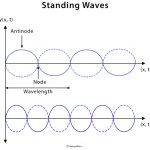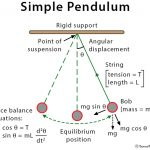Home / Physics / Diverging Lens

# Diverging Lens

## Definition

A lens placed in the path of a beam of parallel rays can be called a diverging lens when it causes the rays to diverge after refraction. It is thinner at its center than its edges and always produces a virtual image.

A lens with one of its sides converging and the other diverging is known as a meniscus lens. A double concave lens is diverging on both of its refracting surfaces. Whereas, if one side has no curvature but the other side is concave, it is known as a plano-concave lens.

## Is Concave Lens a Diverging Lens?

When rays of light pass through a concave lens, they diverge, making the lens work as a diverging lens. So, a concave lens can be considered a diverging lens when operating in the medium of air.

## Ray Diagram Showing Image Formation by Diverging Lens

A diverging lens ray diagram follows three basic rules:

• Any ray of light that is parallel to the principal axis of the lens will pass through its focal point after refraction.
• Any incident ray of light that passes through the focus of the lens before getting refracted will emerge parallel to the principal axis on refraction.
• A ray of light that is incident on the optical center of the lens will pass through undeviated.

For all positions of the object, the image is erect, smaller in size than the object and formed within the focal length of the lens. While drawing a ray diagram, it is essential to consider at least two principal rays emanating from the top of an extended object. While one of them should remain parallel to the principal axis, another one should pass through the optical center of the lens.

## Converging vs. Diverging Lens

The major details are enlisted in the below table.

 Converging Lens Diverging Lens 1. It is thicker at the middle but thinner at the edges It is thinner at the middle but bulging near the boundaries 2. It has a focusing action It diverges a beam of light 3. It can produce both real and virtual images depending on the position of the object It produces a virtual image for all positions of the object 4. It can produce both diminished and magnified images depending upon the position of the object It always produces diminished images irrespective of the position of the object 5. Also known as a positive lens or convex lens Also known as a negative lens or concave lens 6. Image may be inverted or upright Image is always upright

Other than the basic structural variations between concave mirrors and diverging lenses, the main difference  lies in the fact that the former works by the principle of reflection while the latter utilizes that of refraction. Needless to mention, the image formation procedure is completely distinct for the two.

## Diverging Lens Equations

The below lens equation is a quantitative expression of the relationship between the object distance (do), the image distance (di) and the focal length (f) of a thin lens.The focal length of a thick lens in air can be calculated by the lensmaker’s equation,]

where f is the focal length of the lens, n is the refractive index of the material that makes up the lens, R1 is the radius of curvature of the lens surface closest to the object, R2 is the radius of the lens surface farthest from the object and d is the thickness of the lens.  f is positive for a converging lens and negative for a diverging one.

For a thin lens placed in air, d ≈ 0. Hence, the lensmaker’s formula takes the form,The reciprocal of the focal length is known as the optical power of the lens. If the focal length is expressed in meters, then the said quantity determines the optical power in dioptres.

The magnification equation relates the object and image distances with the object height (ho) and image height (hi). According to it, the magnification M produced by a lens is given by,

M =## Applications

Nearsighted, short sighted or myopic people can see nearby objects clearly but have a problem in viewing distant objects as the image is formed before the retina causing it to be blurred. A concave lens can correct the vision by diverging the rays of light emanated from distant objects which are the converged by the eye lens to form a distinct image in the retina.

Refracting telescopes generally use a convex-concave lens combination to enable magnified viewing of far away objects.

The study of the diverging lens has advanced the Optics branch of Physics in a multitude of ways. While it provides an interesting opportunity to learners for grasping the basic characteristics of lenses, it finds ample use in the field of medical science and astronomy as well.

Article was last reviewed on Tuesday, December 10, 2019

### Related articlesStanding WavesFundamental FrequencySimple PendulumKirchhoff’s Law